Next: The Radiopulsar PSRB1259-63Ā- a Up: Ejectors in Massive Binary Previous: Caverns in Binary Systems

The Effect of Relativistic Wind on Accretion Flow Parameters

Now let us try to take into account the rearrangement of the surrounding medium under the action of pulsar radiation in a similar way to how the stationary shells in the propellerĀ regime are computed. Such an analysis, which was carried out by Davies and Pringle (1981), is an extension of the model proposed by Gunn and Rees (1974) for the Crab nebula.Ā

Suppose that a cavern is formed around an ejecting star (for the sake of simplicity, we assume that the cavern is spherical). We assume that the entire energyemitted by the pulsar is dissipated at the internal wall of the cavern. Obviously, the size of the cavern is of the order of the Schwartzman radius. Near the inner boundary of the shell, the velocity of sound is equal to the maximum possible value:Since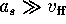the pressure gradient is small and the pressure in the shell is constant. Taking this into consideration, we obtain for the conservation of the energy flux

ĀThe outer radius of the hot shell is determined from the condition:It follows from the second of the solutions (10.5.1) that the ratio of the velocity of sound to the free-fall velocity is. Therefore, as the NS is spun down,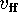becomes of the order of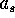first at the outer boundary. Hence, Davies and Pringle proposed that the shell collapses and the ejection stage is terminated when the inequality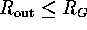is satisfied. This condition is equivalent to the following inequality for the period:

Ā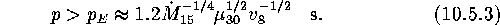If, however, the radius of the light cylinder is larger than the capture radius, i.e., the condition for the termination of the ejection stage remains valid. Equation (10.5.3) can be used if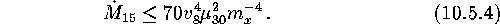Thus, consideration of the inverse effect of pulsar radiation on the properties of accreting plasma makes the ejection possible even when the Schwartzman radius is smaller than the capture radius. It can be seen that in this case the critical period estimation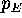is not changed significantly. This is primarily due to the fact that the power of a pulsar, and hence the pressure of the pulsar wind, depends very strongly on its period (as). However, it remains unclear whether the shell will be stable when its inner radius becomes smaller than the capture radius.

Lipunov and Prokhorov (1984) proposed a mechanism for the formation of radiobursts in the case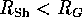which takes into account the possible contribution of pressure of the accumulated magnetic dipole radiation. In this case, the process becomes time-dependent.

Let us assume that the magnetic dipole radiation reflected by the cavern walls is stored inside and prevents the cavern from collapse. If the cavern radius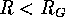, the condition at its boundary can be written as

Ā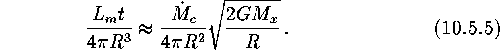It can be seen that the pressure of the radiation in such a cavern rises more rapidly (as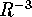) than the plasma pressure (which increases as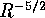). The cavern is filled up very quickly by radiation and begins to expand (as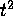). When its radius becomes equal to the capture radius, the gravitational force is no longer important. The size of the cavern increases slightly, it is detached from the NS and drifts upward with the stellar wind emanating from the normal component. Apart from magnetic dipole radiation, the cavern may also contain relativistic particles (which are also reflected from the cavern boundary). Such caverns must produce very short radiopulses when floating and bursting. The total energy and the characteristic radioburst recurrence time can be easily estimated by using equation (10.5.5) for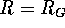. The energy of a radioburst is given by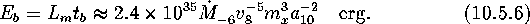The recurrence time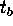and the durationof a burst are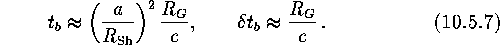ForĀcm and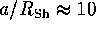-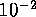,varies from a few hundred seconds to several weeks.Next: The Radiopulsar PSRB1259-63Ā- a Up: Ejectors in Massive Binary Previous: Caverns in Binary Systems

Mike E. Prokhorov
Sat Feb 22 18:38:13 MSK 1997